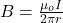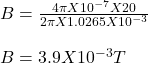## Number 12 gauge wire, commonly used in household wiring, is 2.053mm in diameter and can safely carry up to 20A. For a wire carrying the maxi

Question

Number 12 gauge wire, commonly used in household wiring, is 2.053mm in diameter and can safely carry up to 20A. For a wire carrying the maximum current, find the magnetic field strength:

in progress 0
5 months 2021-09-05T06:58:19+00:00 1 Answers 0 views 0

## Answers ( )

The magnetic field strength is 3.9 x 10⁻³ T

Explanation:

The equation for the magnetic field strength produced by a long straight current-carrying wire is written as;Where;

μ₀ is constant = 4π x 10⁻⁷ N/A

I is the maximum current = 20 A

r is the radius of the wire = 2.053/2 = 1.0265 mm = 1.0265 x 10⁻³ m

Substitute in this values into the equation above;Therefore, the magnetic field strength is 3.9 x 10⁻³ T